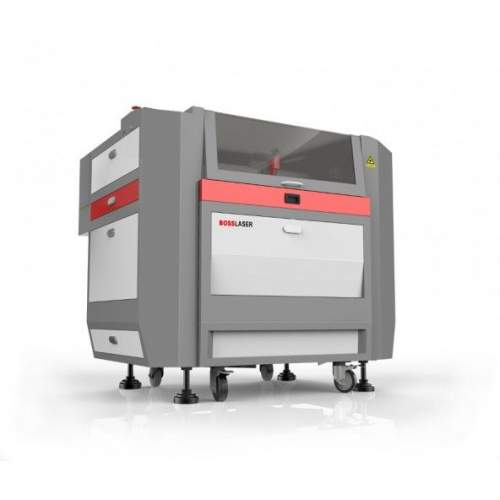# Best Boss Laser Settings for Perfect Results (2021)

The skill of effectively designing, cutting, and engraving a material into what a computer screen can display is very inspiring. Also, this skill is now high in demand.

The Boss Laser company offers one of the best laser cutting and engraving systems/machines. Its sheet metal, interface, overall build, and enclosure are superb! However, using Boss Laser for the first time could be a bit tricky. There are some settings you must apply for perfect results.

This article focuses on how users of Boss Laser can achieve good results via the settings. Whether you are new to industrial fiber laser technology or you already have some experience, this guide will be useful. Keep reading.## Best Settings for Boss Laser Machines

Below are some of the settings for Boss Laser cutting and engraving machines.

To cut Acrylic, use any of these settings:

• For 65 WATTS: Power = 80%, Speed = 10mm/s, Thickness = 1/4″.
• For 100 WATTS: Power = 75%, Speed = 15mm/s, Thickness = 1/4″.
• For 150 WATTS: Power = 65%, Speed = 20mm/s, Thickness = 1/4″.

To engrave Acrylic, use any of these:

• For 65 WATTS: Power = 25%, Speed = 350mm/s, Interval/Scan Gap = .065mm, Thickness = Use any.
• For 100 WATTS: Power = 20%, Speed = 350mm/s, Interval/Scan Gap = .065mm, Thickness = Any.
• For 150 WATTS: Power = 15%, Speed = 350mm/s, Interval/Scan Gap = .065mm, Thickness = Use any.

To engrave Balsa Wood:

• For 65 WATTS: Power = 25%, Speed = 350mm/s, Interval/Scan Gap = .065mm, Thickness = Use any.
• For 100 WATTS: Power = 20%, Speed = 350mm/s, Interval/Scan Gap = .065mm, Thickness = Use any.
• For 150 WATTS: Power = 15%, Speed = 350mm/s, Interval/Scan Gap = .065mm, Thickness = Use any.

To cut Balsa Wood, use any of these settings:

• For 65 WATTS: Power = 60%, Speed = 25mm/s, Interval/Scan Gap = Ignore, Thickness = 1/8″.
• For 100 WATTS: Power = 55%, Speed = 25mm/s, Interval/Scan Gap = Ignore, Thickness = 1/8″.
• For 150 WATTS: Power = 40%, Speed = 25mm/s, Interval/Scan Gap = Ignore, Thickness = 1/8″.

To engrave Anodized Aluminum:

• For 65 WATTS: Power = 35%, Speed = 325mm/s, Interval/Scan Gap = .065mm, Thickness = Use any.
• For 100 WATTS: Power = 20%, Speed = 325mm/s, Interval/Scan Gap = .065mm, Thickness = Use any.
• For 150 WATTS: Power = 15%, Speed = 325mm/s, Interval/Scan Gap = .065mm, Thickness = Use any.

To cut Bass Wood:

• For 65 WATTS: Power = 60%, Speed = 25mm/s, Interval/Scan Gap = Ignore, Thickness = 1/8″.
• For 100 WATTS: Power = 55%, Speed = 25mm/s, Interval/Scan Gap = Ignore, Thickness = 1/8″.
• For 150 WATTS: Power = 40%, Speed = 25mm/s, Interval/Scan Gap = Ignore, Thickness = 1/8″.

To engrave Bass Wood:

• For 65 WATTS: Power = 35%, Speed = 350mm/s, Interval/Scan Gap = .065mm, Thickness = Use any.
• For 100 WATTS: Power = 25%, Speed = 350mm/s, Interval/Scan Gap = .065mm, Thickness = Use any.
• For 150 WATTS: Power = 20%, Speed = 350mm/s, Interval/Scan Gap = .065mm, Thickness = Use any.

To engrave Glass:

• For 65 WATTS: Power = 27%, Speed = 325mm/s, Interval/Scan Gap = .065mm, Thickness = Use any.
• For 100 WATTS: Power = 25%, Speed = 325mm/s, Interval/Scan Gap = .065mm, Thickness = Use any.
• For 150 WATTS: Power = 20%, Speed = 325mm/s, Interval/Scan Gap = .065mm, Thickness = Use any.

These are some of the settings to achieve perfect results with Boss Laser’s cutters and engravers. For more settings of other materials, please visit the official website: Boss Laser Settings.